Next Article in Journal
A „Hybrid“ Thin-Film pH Sensor with Integrated Thick-Film Reference
Previous Article in Journal
Evaluation of Protein Adsorption on Chitosan Surfaces with Reflectometry Interference Spectroscopy
Article

# Sensors and Asymptotic w-observer for Distributed Diffusion Systems

University of Perpignan, Systems Theory Laboratory, 52, Avenue de Villeneuve, 66860 Perpignan, France
*
Author to whom correspondence should be addressed.
Sensors 2001, 1(5), 161-182; https://doi.org/10.3390/s10500161
Received: 18 July 2001 / Accepted: 16 August 2001 / Published: 27 October 2001

## Abstract

The aim of this paper is to study the regional observer concept through the consideration of sensors. For a class of distributed diffusion systems, we propose an approach derived from the Luenberger observer type as introduced by Gressang and Lamont . Furthermore, we show that the structures of sensors allow the existence of regional observer and we give a sufficient condition for each regional observer. We also show that, there exists a dynamical system for diffusion systems is not observer in the usual sense, but it may be regional observer.
Keywords:

## Introduction

The observability problems in distributed systems have been the subject of a good deal of research [2,3,4,5,6,7]. These systems are the general representation of several physical systems described by partial differential equations or differential equations [8,9]. In this paper, we are concerned with some methods of construction of an asymptotic regional state for infinite dimensional systems described, in terms of linear semi-group. The notion of asymptotic regional construction has been introduced by Al-Saphory and El Jai  and is based on the concept of regional detectability . In this work, we develop this approach and the mathematical tools which allow to construct various types of ω-observers in a given subregion ω of the domain Ω, in connection with sensors structures. The results are considered in a particular case of parabolic systems. The principal reason behind introducing this concept is that it provides a means to deal with some physical problems concern the determination of laminar flux conditions, developed in steady state by vertical uniformly heated plate (Fig. 1).
Figure 1. Profile of the active plate.
Figure 1. Profile of the active plate.This approach can be extended to find the unknown boundary convective condition on the front face of the active plate, as in . The reconstruction is based on knowledge of the dynamical system (ω-observer) and the measurement given by internal pointwise sensors (that means by the thermocouples).
The paper is organized as follows. Section 2 is devoted to the presentation of the system under consideration and preliminaries. We recall that the definitions of ω-stability and ω-detectability and we also give the definitions of different types of ω-observers (case general, identity and reduced-order). Section 3, we characterize each ω-observers in terms of sensors structures and we give a counterexample of the case ω-observer is not observer in the whole domaine Ω. The useful applications of these results are considered.

## 2. Asymptotic ω-observer

#### 2.1 Description systems and preliminaries

Suppose (SA(t))t≥0 an exponentially stable, strongly semi-group of operators on the space X, with generator A : D(A) → X may be linear differential elliptic defined by
Ax(ξ,t) = Δx(ξ,t)
We denote $Q$=Ω×(0, ∞), Θ=∂Ω×(0, ∞) and we consider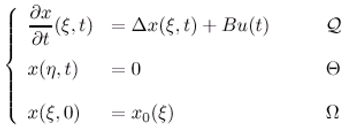and measurements are given by the output function
$z ( t ) = C x ( ξ , t )$
where the following hypothesis are considered.
• The operators Δ is self-adjoint with compact resolvent.
• Ω is an open regular bounded set of $R n$ (n =1, 2, 3) with smooth boundary ∂Ω.
• ω is a nonempty given subregion of Ω.
• X, U, $O$ are separable Hilbert spaces where X is the state space, U the control space and $O$ the observation space and with X = L2 (Ω), U = L2 (0, ∞; $R q$) and $O$ = L2 (0, ∞ ; $R p$).
• The operators B : UX and C : X$O$ are bounded linear and depend on the structure of actuators and sensors . Under the above assumption, the system (2.1) has a unique solution given by
$x ( ξ , t ) = S A ( t ) x 0 ( ξ ) + ∫ 0 t S A ( t − s ) B u ( s ) d s$
The problem consists to construct a regional observer which gives an estimated state of x(ξ, t) in ω by using only the measurements (2.2). We recall that:
A sensor may be defined by any couple (D, g) where :
• D denotes a closed subset of $Ω ¯$ , which is spatial support of sensors,
• gL 2 (D) defines the spatial distribution of measurements on D.
According to the choice of the parameters D and g, we have various types of sensors. A sensors may be of zone types when D ⊂ Ω. The output function (2.2) can be written in the form
$z ( t ) = C x ( ξ , t ) = < x ( . , t ) , g i ( . ) > L 2 ( D )$
A sensor may also be a pointwise when D = {b) and g = δ(. – b) where δ is the Dirac mass concentrated in b. Then the output function (2.2) may be given by the form
$z ( t ) = C x ( ξ , t ) = x ( b , t )$
In the case of boundary zone sensor, we consider D = Γ with Γ ⊂ ∂Ω and g L2 (Γ). The output function (2.2) can then be written in the form
$z ( t ) = C x ( ξ , t ) = < x ( . , t ) , g ( . ) > L 2 ( Γ ) .$
The operator C is unbounded and some precautions must be taken in .
• The function χω is defined bywhere x |ω (ξ, t) is the restriction of the state x(ξ, t) to ω. Define now the operator
K : xXKx = CSA(t)x$𝒪$
then z(t)=K(t)x0(.). We denote by K* : OX the adjoint of K given by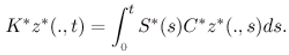The autonomous system associated to (2.1)-(2.2) is exactly (respectively weakly) ω-observable if :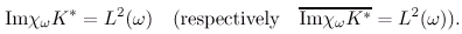The suit of sensors (Di,gi)1 ≤ i ≤ q is ω-strategic if the system (2.1)-(2.2) is weakly ω-observable . The concept of ω-strategic has been extended to the regional boundary case as in [13-15].

#### 2.2 ω-observer

The theory of observer was introduced by Luenberger  and has been generalized to systems described by semi-group operators . Recently, these results have been extended to the regional case by Al-Saphory and El Jai . This extension is based on the concept of regional detectability. In this section, we define the asymptotic ω-observer in a given subregion ω.
Definition 2.1. The system (2.1) is said to be ω-stable, if the operator A generates a semi-group which is stable on L2(ω). It is easy to see that the system (2.1) ω-stable, if and only if, for some positive constants M and α, we have
||χωSA(t)||L2(ω)ME−αt,  ∀t ≥ 0
If (SA(t))t≥0 is stable semi-group on L2(ω), then for all x0L2 (Ω), the solution of the associated autonomous system satisfiesDefinition 2.2. The system (2.1) together with the output function (2.2) is said to be ω-detectable if there exists an operator Hω : $O$L2 (ω) such that (AHωC) generates a strongly continuous semigroup (S(t))t≥0 which is stable on L2(ω).
Definition 2.3. Suppose that there exists a dynamical system with state y(ξ, t) ∈ Y (a Hilbert space) given bywhere Fω generates a strongly continuous semi-group (S (t))t≥0 which is stable on the Hilbert space Y, Gω$L$ (U, Y) and Hω$L$ ($O$ ,Y). The system (2.11) defines an ω-estimator for χωTx(ξ,t) if:
• $lim t → ∞$[y(ξ,t)−χωTx(ξ,t)] = 0, ξω
• χωTx maps D(Δ) into D(Fω) where x(ξ, t) and y(ξ, t) are the solutions of (2.1) and (2.11).
Definition 2.4. The system (2.11) is ω-observer for the system (2.1)-(2.2) if it satisfies the following conditions:
• There exists R$L$ ($O$ ,L2 (ω)) and S$L$ (L2 (ω)) such that RC + ωT = Iω
• χω - FωχωT = HωC and Gω = χωTB.
• The system (2.11) determines ω-estimator for χωTx(ξ, t).
The purpose of ω-observer is to provide an approximation to the state of the original system. This approximation is given by
$x ^ ( ξ , t ) = R z ( t ) + S y ( ξ , t )$
It is clear that:
The system (2.11) is said to be an identity ω-observer for the system (2.1)-(2.2) if χωT=Iω and X=Y.
The system (2.11) is said to be a reduced-order ω-observer for the system (2.1)-(2.2) if X=$O$ ⊕Y.

## 3. Sensors and ω-observer reconstruction

In this section, we give an approach which allows to construct an ω-estimator of Tx(ξ,t). This method avoids the calculation of the inverse operators  and the consideration of the initial state , it enables to observe the current state in ω without needing the effect of the initial state of the original system. Let us consider the set (ϕnj) of eigenfunctions of L2(Ω) orthonormal in L2(ω) associated with the eigenvalues λn of multiplicity rn and suppose that the system (2.1) has unstable modes.

#### 3.1 General case

The problem of asymptotic ω-observability may be studied through the observation operator C. That means, we can characterize the ω-observer by a good choice of the sensors. For that objective, suppose that information is retrieved from the system by p sensors (Di, gi)1≤i≤p. In the asymptotic regional state reconstruction various types of sensors can be considered (Fig. 2).
Figure 2. The estimated state in ω and various sensor locations.
Figure 2. The estimated state in ω and various sensor locations.#### 3.1.1 Case of pointwise sensors

Consider again the systemIn this case the output function (2.2) is given by
$z ( t ) = C x ( . , t ) = x ( b i , t )$
where bi∈Ω is the sensor locations. Let ω be a given subdomain of Ω and assume that for T$L$ (L2(Ω)), and χωT there exists a system with state y(ξ, t) such that
$y ( ξ , t ) = χ ω T x ( ξ , t )$
where χω is defined in (2.7) and we denote Tω =χωT. The equations (3.2)-(3.3) give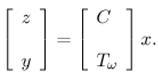If we assume that there exist two linear bounded operators R and S where R : $O$L2(ω) and S : L2(ω)L2(ω), such that RC+STω = I, then by deriving y(ξ,t)in (3.3) we have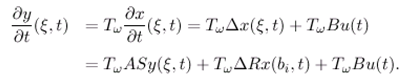Consider now the system (which is destined to be the ω-observer)where Fω generates aa strongly continuous semi-group (S(t))t≥0 which is assumed to be stable on L2(ω), i.e.and Gω$L$ (U, L2(ω)) and Hω$L$ ($O$, L2(ω)). The solution of (3.5) is given byThe problem is that, how to observe asymptotically the current state in ω, i.e. to show that under convenient hypothesis, the state of the system (3.5) is an estimator of Tωx(ξ, t).
Theorem 3.1. Suppose that the operator Fω generates a strongly continuous semi-group which is stable on L2(ω), then the system (3.5) is ω-observer for (3.1)-(3.2), that is,if the following conditions hold:
• There exist R$L$ ($O$, L2(ω)) and S$L$ (L2(ω)) such that
$R C + S T ω = I$
•Proof: For y(ξ, t) = Tωx(ξ, t) and $y ^$(ξ,t) solution of (3.5), denote e(ξ,t) = y(ξ,t)−$y ^$(ξ,t). We haveThus e(ξ,t) = SFω(t)e(0,t) where e(0,t) = Tωx0(ξ) − $y ^ 0$(ξ). Now the stability of the operator Fω leads totherefore $lim t → ∞$e(ξ,t) = 0 . Let $x ^$(ξ, t) = Rx(bi, t)+ S $y ^$(ξ,t), then we haveFinally we have $lim t → ∞ x ^$(ξ,t) = x(ξ,t). ■
From this theorem, we can deduce the following statements:
• This conditions (3.6) and (3.7) in theorem 3.1 guarantee that the dynamical system (3.5) is ω-observer for the system (3.1)-(3.1).
• A system which is an observer is ω-observer.
• If a system is ω-observer, then it is ω1-observer in every subset ω1 of ω, but the coverse is not true. This may be proven in the following example:
Example 3.2. Consider the systemaugmented with the output functionwhere γ1 > 0, γ2 > 0, a > 0, Ω = ]0,a[ and b ∈ Ω is the location of the sensor (b,δb). The operatorgenerates a strongly continuous semi-group (SA(t))t0 on the Hilbert space L2(Ω). Consider the dynamical system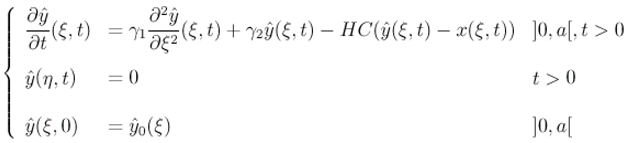where H$L$ ($O$,Y), Y is a Hilbert space and C : Y$O$ is a linear operator. If b/a ∈ ∩ [0, a], then the sensor (b, δb) is not strategic for the unstable subsystem of (3.8) and therefore the system (3.8)-(3.9) is not detectable in Ω. Then, the dynamical system (3.46) is not observer for the system (3.8)-(3.9) . We consider the region ω = [α,β] ⊂ [0,a] (Fig. 3) and the dynamical system.
Figure 3. The domain Ω, the subregion ω and the sensor location b.
Figure 3. The domain Ω, the subregion ω and the sensor location b.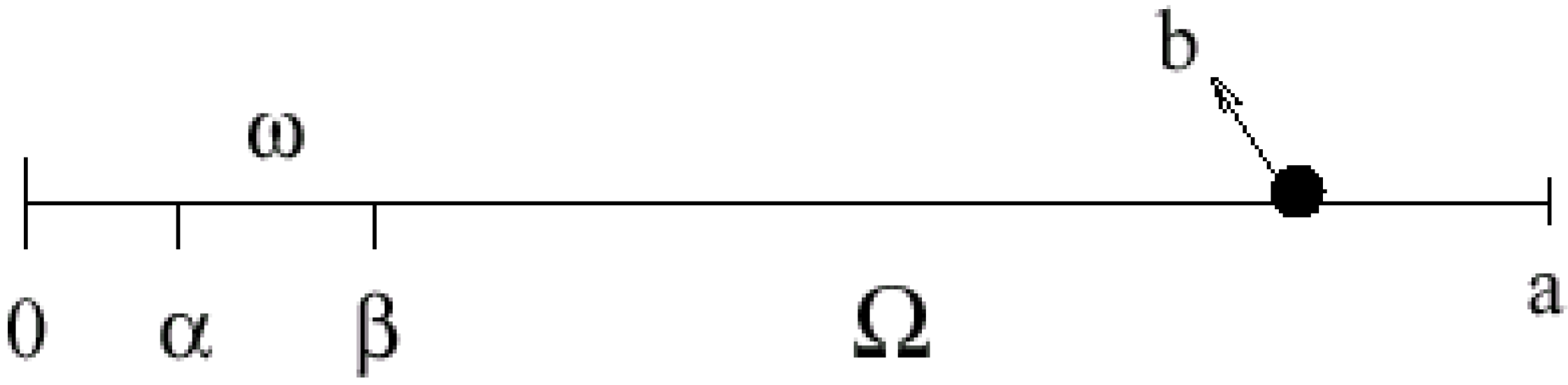where Hω$L$ (L2($O$, ∞, $R q$), L (ω)). If b/a ∉ IQ ∩ ]0,a[, then the sensor (b, δb) is ω-strategic for the unstable subsystem of (3.8)  and therefore the system (3.8)-(3.9) is ω-detectable , i.e.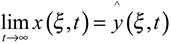. Finally the dynamical system (3.11) is ω-observer for the system (3.8)-(3.9).

#### 3.1.2 Case of zone sensors

In this case, we consider the system (3.1) with the output function (2.4) and we assume that there exists an operator Tω is such that
y (ξ,t) = Tωx(ξ,t)
So, regional observer may be described bywith solution is represented by the equationThus, we obtain the following proposition:
Proposition 3.3. If following conditions hold :
• The operator Fω generates a strongly continuous semi-group which is stable on the space L2(ω).
• There exist $R$$L$ ($O$, L2 (ω)) and S ∈ $L$ (L2(ω)) such that
RC + STω = I
•Then, the dynamical system (3.12) is ω-observer for (3.1)-(2.4).

#### 3.1.3 Case of boundary sensors

Here, we consider the system (3.1) augmented with output function (2.6). The related dynamical system may be expressed by the formThe solution of (3.13) is given byTherefore we have the following result:
Proposition 3.4. Under the following conditions :
• The operator Fω generates a strongly continuous semi-group which is stable on the space L2 (ω).
• There exist $R$$L$ ($O$, L2 (ω)) and S ∈ $L$ (L2(ω)) such that
RC + STω = I
•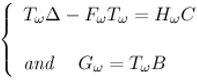the dynamical system (3.13) is ω-observer for (3.1)-(2.6).

#### 3.2 Identity ω-observer

In this case, we consider Tω = I and Z = X, and so the operator equation TωΔ - FωTω = HωC of the regional observer reduces to Fω = Δ - HωC where A and C are known. Thus, the operator Hω must be determined such that the operator Fω is stable. In the case where the sensors are zone types, we consider the system (3.1)-(2.4), together with the dynamical system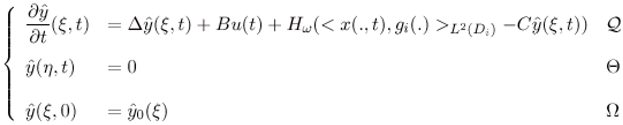Thus the sufficient condition of an identity ω-observer (Fig. 4), is formulated in the following proposition :
Proposition 3.5. If there exists a suite of p zones sensors (Di, gi)1≤ i≤ p which is ω-strategic for unstable subsystem of the system (3.1)-(2.4), then the dynamical system (3.14) is an identity ω-observer for (3.1)-(2.4).
Proof: Let us denote. Then by deriving $e ¯$(ξ,t), we getSince the sensors (Di, gi)1≤ i≤ p are regionally strategic for unstable subsystem of (3.1)-(2.4), the subsystem is weakly ω-observable, and since it is finite dimensional, then it is exactly ω-observable. Therefore it is ω-detectable , there exists an operator $L$ (L2(0, ∞, $R q$), L2(ω)), such that (Δ-HωC) generates a strongly continuous, stable semi-group (S(t))t≥ 0 on the space L2(ω) which is satisfied the following: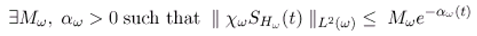Finally, we haveand therefore $e ¯$(ξ,t) → 0 when t → ∞ . In this case, the operators R = 0 and S = I with $x ^$(ξ,t) = $y ^$(ξ,t), then we haveThis lead to $lim t → ∞ x ^$(ξ,t) = x(ξ,t). Then, the system (3.14) is an identity ω-observer for (3.1)-(2.4).
In the case where the sensors are pointwises, we consider the system (3.1)-(2.5) with the corresponding dynamical systemmay be considered as an identity ω-observer. So, we have the following result:
Proposition 3.6. If there exists a suite of p pointwise sensors (bi, δbi)1≤ i≤ p which is ω-strategic for unstable subsystem of the system (3.1)-(2.5), then the dynamical system (3.15) is an identity ω-observer for (3.1)-(2.5).
In the case where the sensors are boundary zones, we also consider the system (3.1) together with output function (2.6). Then the system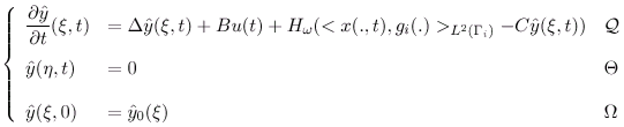may be consider as ω-observer for (3.1)-(2.6). Then we have the following result:
Figure 4. Identity ω-observer.
Figure 4. Identity ω-observer.Proposition 3.7. If there exists a suite of p boundary zones sensors (Γi, gi)1 ≤ i ≤ p which is ω-strategic for unstable subsystem of the system (3.1)-(2.6), then the dynamical system (3.16) is an identity ω-observer for (3.1)-(2.6).

#### 3.3 Application to an identity ω-observer in diffusion system

Consider the case of two dimensional system defined in Ω =]0, 1[ × ]0, 1[ by the parabolic equationand suppose there is only one boundary zone sensor (Γ, g) located on Γ = [ηO1 - l1, 1] × {0} ∪ {1} × [0, ηO2 + l2] ⊂ ∂Ω. The sensor (Γ, g) may be sufficient for the measurement part of the desired state . In this case the output function is given byand the considered subregion ω =]α1, β1[ × ] α2, β2 [⊂ Ω =]0, 1[ × ]0, 1[, (see Fig. 5).
The eigenfunctions related to the operator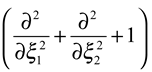are given byassociated with eigenvalues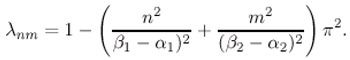Figure 5. The domain Ω, the subregion ω and the sensor location Γ.
Figure 5. The domain Ω, the subregion ω and the sensor location Γ.By applying the proposition 3.7, the system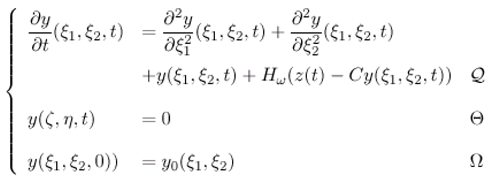is an identity ω-observer for the system (3.17)-(3.18) ifThis leads to (ηO1 – α1) / ( β1 – α1) and (ηO2 – α2) / ( β2 – α2) ∈ $N$ for every n, m =1, ... ,J, and hence the boundary sensor (Γ, g) is ω-strategic.

#### 3.4 Reduced-order ω-observer

In the case where the output function (2.2) gives information about a part of the state vector x(ξ,t), it is necessary to define an asymptotic observer enables to construct the unknown part of the state. Consider now X = X1X2 where X1 and X2 are subspaces of X. Under the hypothesis of section 2, the system (2.1) can be decomposed by:where x1X1, x2X2, B1$L$ (Z1, U) and B2$L$ (X2, U). Using the decomposition of (3.20), the system (3.1) can be written by the form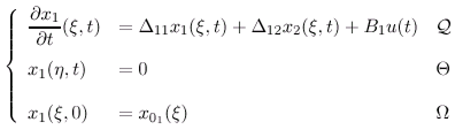and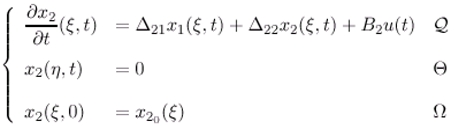Now we discuss this problem with various sensors. Thus in the case of pointwise sensors the system (3.21)-(3.22) augmented with output function.
z(t) = x1(bi, t)
In this section, the problem consists in constructing ω-observer which enables to estimate the unknown part x2 (ξ,t) . Equivalently, the problem is reduced to define an identity ω-observer for the system (3.22). The equations (3.22)-(3.23) allow the following system.with the output functionFrom the proposition 3.6, we have an identity ω-observer for the system (3.24)-(3.25) given byIf the sensors (Di, gi)1≤ i ≤ p are ω-strategic for the subsystem (3.21)-(3.23) and this result gives the following relation:where sup rn = r < ∞ and j =1,..., rn. Thus we have the following theorem:
Theorem 3.8. If the sensors (Di, gi)1 ≤ i ≤ p are ω-strategic for the unstable subsystem (3.21)-(3.23), then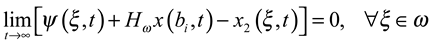where x(bi, t) is the output of the initial system and w is the solution of equationProof: The solution of the identity ω-observer (3.26) is given byFrom the equations (3.21) and (3.25), we haveInserting (3.30) into (3.29), we obtainand we can getUsing Bochner integrability properties and closeness of (A22 - HωΔ12), the equation (3.32) becomes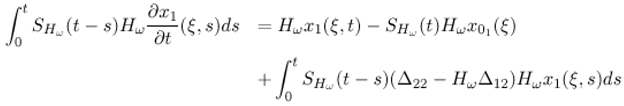Substituting (3.33) into (3.31), we have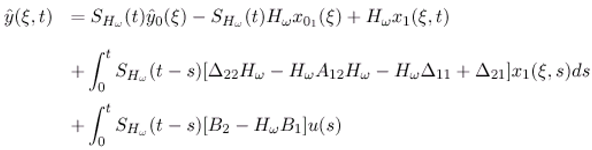Setting ψ(ξ,t) = $y ^$(ξ,t)+ Hωx(b,t), with ψ0(ξ) = $y ^ 0$+ Hωx01 (ξ), where z0(ξ) = x01 (ξ). Now, assume that (Δ22HωHωΔ12HωHωΔ11 + Δ21) and (B2HωB1) are strongly continuous, the equation (3.34) can be differentiated to yield the following systemand thereforeFrom the relation (3.27) and (3.28), we can deduce that the system (3.24)-(3.25) is ω-detectable , there exists an operator Hω$L$ ($O$, L2(ω)), such that (A22HωA12) generates a stable semi-group (SHω (t))t≥0 on the space L2 (ω):Thus we obtainIn this case, $x ^$(ξ,t) = y2(ξ,t), then we haveand henceThen, from this result, we have:
• The state vector $y ^ 2$(ξ,t) can be represented bywhich estimates asymptotically the state vector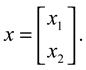2.
The component $y ^ 2$(ξ,t) is an asymptotically estimator of x2(ξ,t).
3.
The system (3.26) is a reduced-order ω-observer for the system (3.24)-(3.25) (Fig. 6).
4.
If we consider X1 = L2 (0, ∞; $R p$) and X2 = Y where Y is the state space for the ω-observer. So, from the theorem 3.8, the reduced-order ω-observer can reconstruct the unknown state components (xp+1,xp+2, ...), thus the condition (3.7) of the theorem 3.1 is satisfied, if we define the following operators as below.and we obtain the relation RC + STω = Iz1.
In the case of zone sensors, we consider the system (3.21)- (3.22) augmented with output functionThe equations (3.22)-(3.36) lead to the following system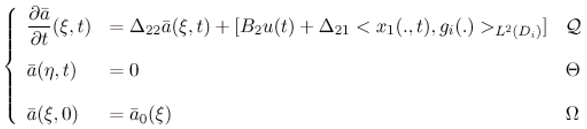with the output functionFigure 6. Reduced-order ω-observer.
Figure 6. Reduced-order ω-observer.Using the proposition 3.5, we have an identity regional observer for the system (3.37)-(3.38) given byIf the sensors (Di, gi)1≤ i ≤ p are ω-strategic for the subsystem (3.37)-(3.38), then we have the relation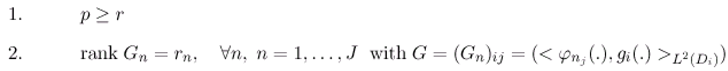where sup rn = r < ∞ and j =1,...,rn. We have the result.
Proposition 3.9. The dynamical system (3.39) is a reduced-order ω-observer, if there existe a suite of p zone sensors (Di, gi)1 ≤ i ≤ p, which is ω-strategic for unstable subsystem of the original system.
The case of boundary pointwise sensors is similar to the case pointwise sensors. In the case of boundary zone sensors, we consider again the equations (3.21) and (3.22) with the output functionwith the output functionFrom proposition 3.7 can be characterized an identity ω-observer for the system (3.41)-(3.42) given by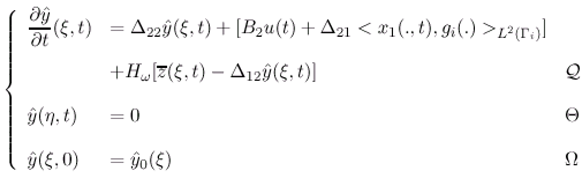If the sensors (Di, gi)1≤ i ≤ p are ω-strategic for the subsystem (3.21)-(3.42) and this result gives the following relationwhere sup rn = r < ∞ and j =1,..., rn.
Proposition 3.10. The dynamical system (3.43) is a reduced-order ω-observer, if there exist a suite of boundary zone sensors (Γi, gi)1≤ i ≤ p which is ω-strategic for unstable subsystem of the original system.
These results can be extended to the case of Neumann boundary conditions.

#### 3.5 Application to a reduced-order ω-observer in diffusion systems

Consider the case of one dimensional system described by the parabolic equationSuppose that information retrieved by p pointwise sensors. The output function is givenwhere Ω =]0, 1[ and (bi)1≤ i ≤ p ∈ Ω are the pointwise sensors locations (Fig. 7).
Figure 7. The domain Ω, the subregion ω and the location bi of the pointwise sensor.
Figure 7. The domain Ω, the subregion ω and the location bi of the pointwise sensor.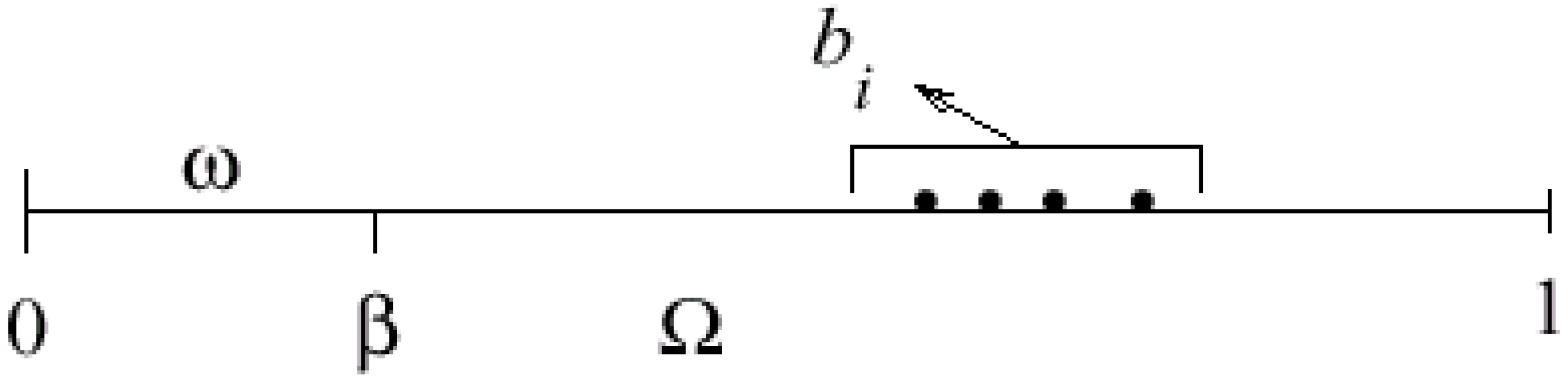The output function (3.45) gives the first p components of the state vector Ψ(ξ,t). Thus the residue componentsmay be constructed asymptotically the second part of the state vector. For this purpose, consider the subregion ω =]0,β[ ⊂ ]0, 1[ and the eigenfunctions of the operator Δ related to ω are defined byIn this case, the operator B = 0, Δ1221 = 0 and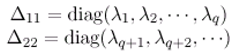The system (3.44)-(3.45) is equivalent to the system (2.1)-(2.2). The associated eigenvalueswith λ1 > λ2 > ... λJ > 0 > λJ+1 > ... . The theorem 3.8 allow to estimate the unknown components Ψ asymptotically where $Ψ ¯$p+1, Ψp+2,.... . . If the sensors areω-strategic for the unstable par of the subsystem with Δ11. That means, the following the relation holds: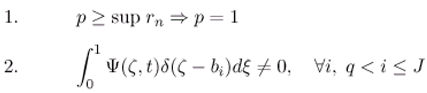then, we obtainξω withand so, for pJ the components of the unstable modes of the output function are required to be non-zero, and for p > J, the sensors are also strategic for the subsystem with Δ11. Finally the dynamical systemis a reduced ω-observer for the system (3.44)-(3.45).

## 4. Conclusion

In this paper we have studied the concept of ω-observer, of a distributed diffusion system whose behavior is expressed in the terms of infinite-dimensional system. More precisely, we have given an extension of asymptotic regional state reconstruction in the considered subregion ω, based on the structures of sensors. Thus, we have characterized the existence of such ω-observer (general case, identity, reduced-order). The case where the state to be asymptotically estimated on a part of boundary of the domain Ω is under consideration.

## References

1. Gressang, R.; Lamont, G. B. Observers for systems characterized by semi-groups. IEEE on Automatic and Control 1975, 20, 523–528. [Google Scholar] [CrossRef]
2. Kitamura, S.; Sakairi, S.; Nishimura, M. Observer for distributed-parameter diffusion systems. Electrical engineering in Japan 1972, 92, 142–149. [Google Scholar] [CrossRef]
3. Hautus, M. L. Strong detectability and observers. Linear Algebra and its Applications 1983, 5, 353–368. [Google Scholar] [CrossRef]
4. El Jai, A.; Berrahmoune, L. Localisation d’actionneurs-zones pour la controlabilite de systemes paraboliques. C. R. Acad. Sc. 1983, 297, 647–650. [Google Scholar]
5. El Jai, A.; Berrahmoune, L. Localisation d’actionneurs ponctuels pour la controlabilite de systemes paraboliques. C. R. Acad. Sc. 1984, 298, 47–50. [Google Scholar]
6. El Jai, A.; Berrahmoune, L. Localisation d’actionneurs-frontiere pour la controlabilite de systemes paraboliques. C. R. Acad. Sc. 1984, 298, 177–180. [Google Scholar]
7. El Jai, A.; Pritchard, A. J. Sensors and controls in the analysis of distributed systems; Ellis Horwood series in Mathematics and its Applications; Wiley: New York, 1988. [Google Scholar]
8. El Jai, A.; Zerrik, E.; Simon, M. C.; Amouroux, M. Regional observability of a thermal process. IEEE Trans. on Automatic Control 1995, 40, 518–521. [Google Scholar] [CrossRef]
9. El Jai, A.; Guisset, E.; Trombe, A.; Suleiman, A. Application of boundary obser-vation to a thermal system. In Conf. of MTNS 2000, Perpignan, France, June 19-23.
10. Al-Saphory, R.; El Jai, A. Asymptotic regional state reconstruction. Int. J. of Systems Science 2000. submitted. [Google Scholar]
11. Al-Saphory, R.; El Jai, A. Sensors structures and regional detectability of parabolic distributed systems. Sensors and Actuators 2001, 29, 163–171. [Google Scholar] [CrossRef]
12. El Jai, A.; Simon, M. C.; Zerrik, E. Regional observability and sensor structures. Sensors and Actuators 1993, 39, 95–102. [Google Scholar] [CrossRef]
13. Al-Saphory, R.; El Jai, A. Sensors characterizations for regional boundary detectability of distributed parameter systems. Sensors and Actuators 2001, 94, 1–10. [Google Scholar] [CrossRef]
• Sample Availability: Available from the author.